# Python Sets

A Set in Python is like the sets you probably studied in Mathematics but with some extra features. There are some unique properties of Python Sets that make them different from other sequential data types like Lists and Tuples, let’s have a look: –

• Operations like Slicing and Indexing cannot be performed on Sets because they are unindexed and unorganised unlike Lists and Tuples. However, mathematical operations like Union and Intersection are supported.
• Set as a whole is mutable, i.e., it can be updated but the elements present in the set are unchangeable/immutable.
• A Set cannot contain a duplicate element of that element.

Python also has also a sequence that is called Frozen Set, it is similar to a Set except for a one property. Frozen Sets are explained later in this article. First, let’s see how you can create a Set.

## Creating a Set

Sets can be created using the set function like this: –

## ExampleOr, you can create a Set by simply placing all the elements in curly braces: –

## Example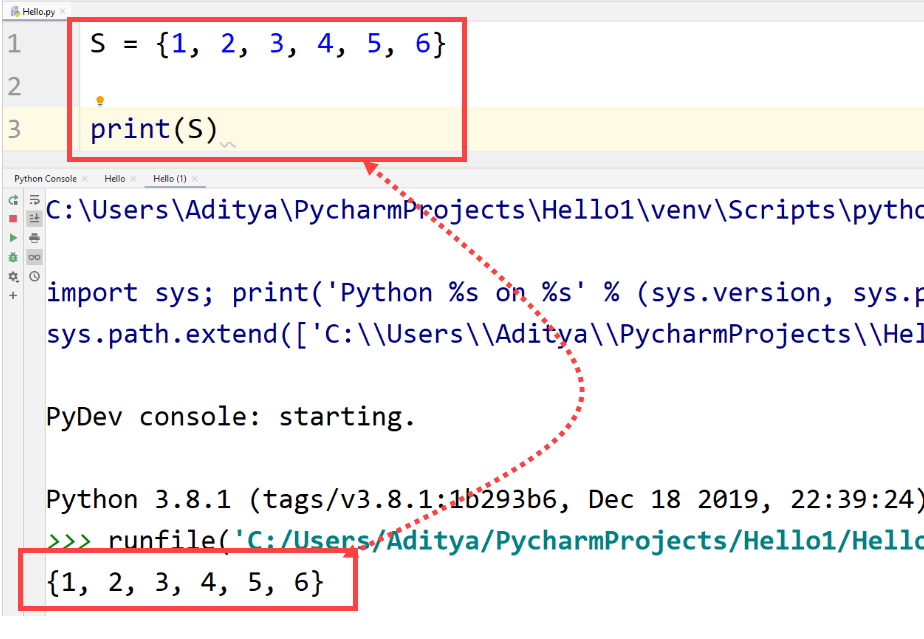Curly braces when used empty are identified as a Dictionary (another sequential data type in Python) in Python. To create an empty set, the method set() is used.

## Example## Updating Sets

It is true that elements in a set cannot be accessed/changed individually but more elements can be added or removed from a Set. This makes a Set immutable sequential data type.

Single element in a Set can be added using add() method. However, if you want to add multiple methods in a Set then you will have to use update() method.

## ExampleAs discussed, let us see how to update multiple elements using the update() method.

## Example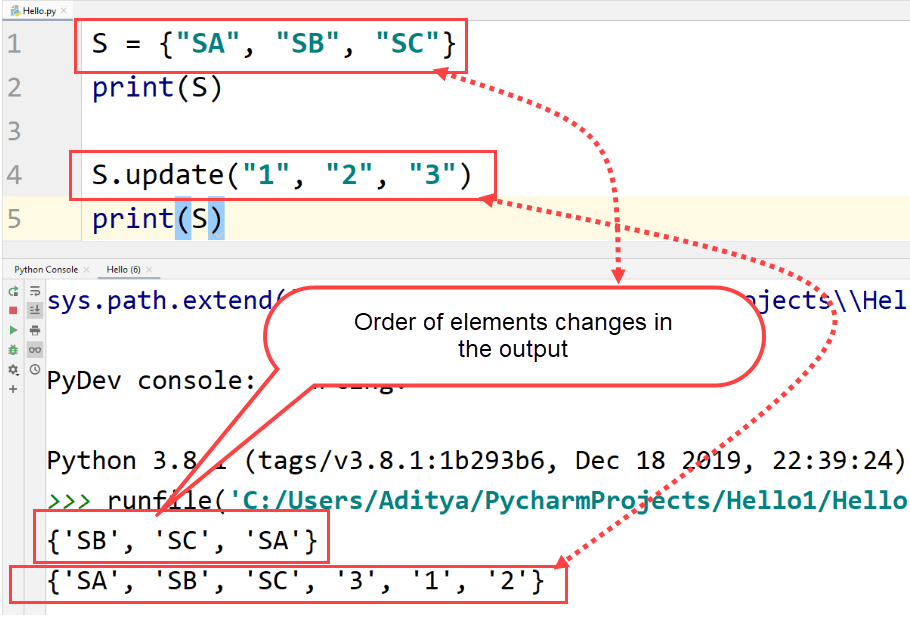Remove Elements

Items can also be removed from a Set by using discard() or remove() method like this: –

## ExampleIf you want to remove a non-existing element, Python interpreter will show an error.
Also, a whole Set can be removed from memory by using the del keyword, printing that Set will give you an error.

## Example## Operation on Sets

Operations on Sets are different from conventional mathematical operations like “+” (Addition), “-” (Subtraction), etc. Operations like union, difference, intersection and symmetric difference can be carried out Sets though.
The syntax uses operators as functions and passes one of the operands as an argument in the function.

## Union

A Union operator returns the elements of both the Sets that are passed as Operands.

## ExampleThe same can be performed using “|” operator.

Here is a mathematical representation of Union of Sets.## Difference

This operator returns the elements that are only present in the left operand. This is carried out using the subtraction (-) sign.

## ExampleHere is a mathematical approach to the difference operator:## Intersection

Intersection operator returns all the elements that are common in both the Sets. It is performed using the & operator. However, this can also be performed using the intersection() operator in the same way as shown in the union of sets.

## Example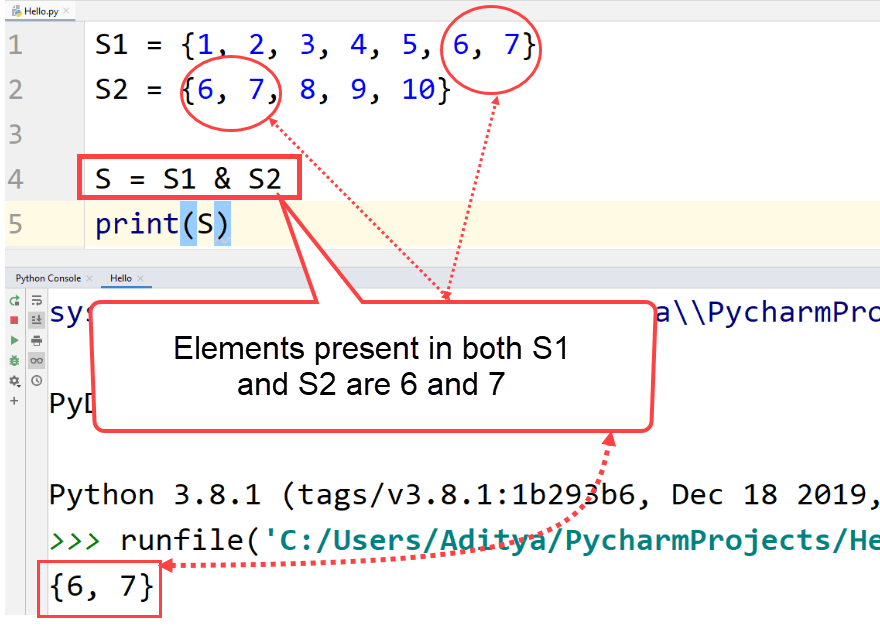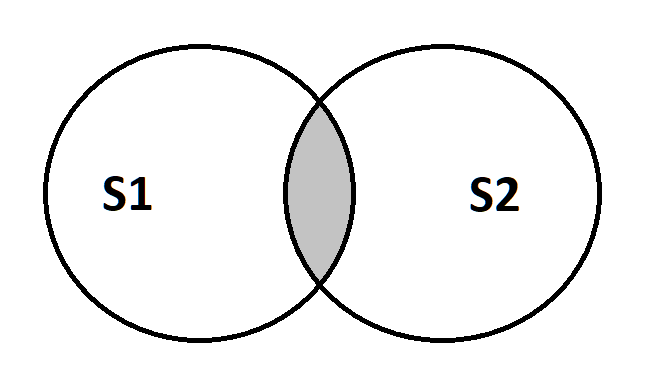This is how you might have learned the intersection of Sets in your Mathematics classes.

## Symmetric Difference

Symmetric difference is just the opposite of Intersection of Sets. It only prints the elements that are not common in both the sets.

Here is the Venn diagram of Symmetric Difference.It is carried out by the operator “^” and can also be done by using the symmetric_difference() method.

## Example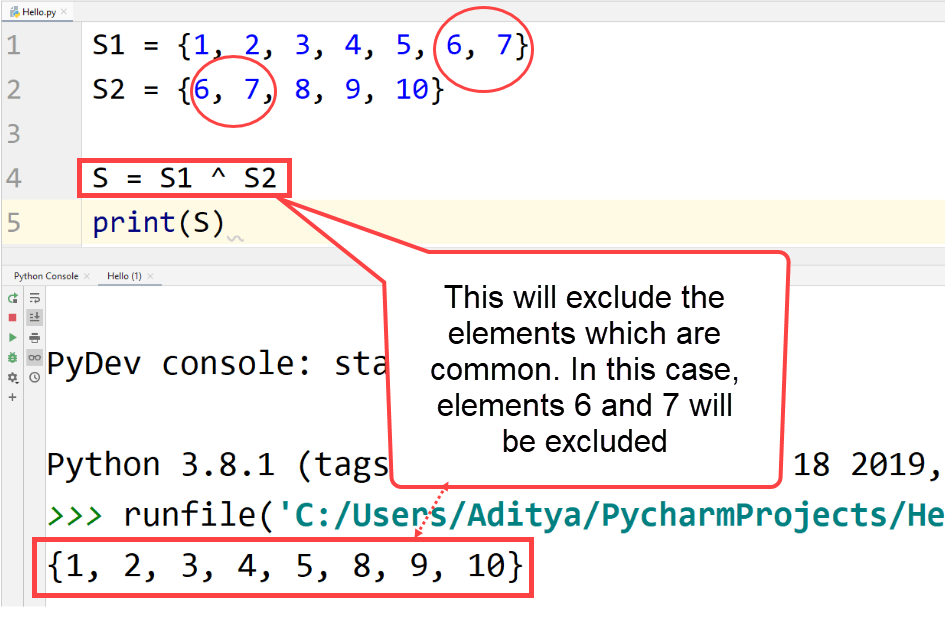## Iterating through a Set

As there are no specific indexes associated to elements, it is not possible to access a single element in a Set. However, we can check if a certain element is present in a Set or not. Also, a Set can be iterated using a “for” loop.

## Example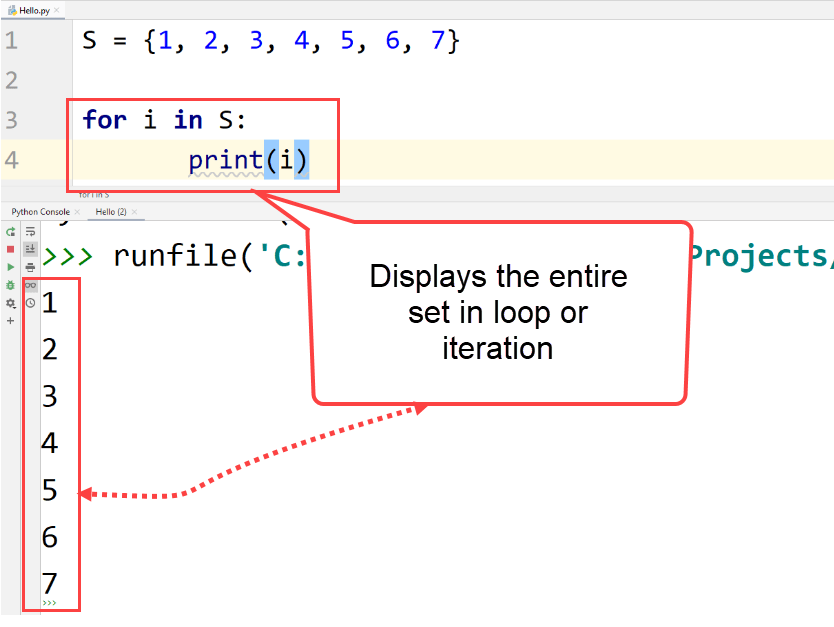To check whether an element is present in a Set or not, this can be done

## ExampleIf the value entered is present in the Set, then the output will be True, else it will be False. For the above example, the output will be False.

## Frozen Sets

Frozen Sets are just like regular Sets except that the Fronzenset objects cannot be changed, i.e., Frozen Sets are immutable. We can also say that Tuples are Frozen Lists.

The function to use Frozen set is – frozenset()

## ExampleAs you can see Frozen Sets print just like regular sets but when we try to change their values then the Python interpreter gives you an error.

## Example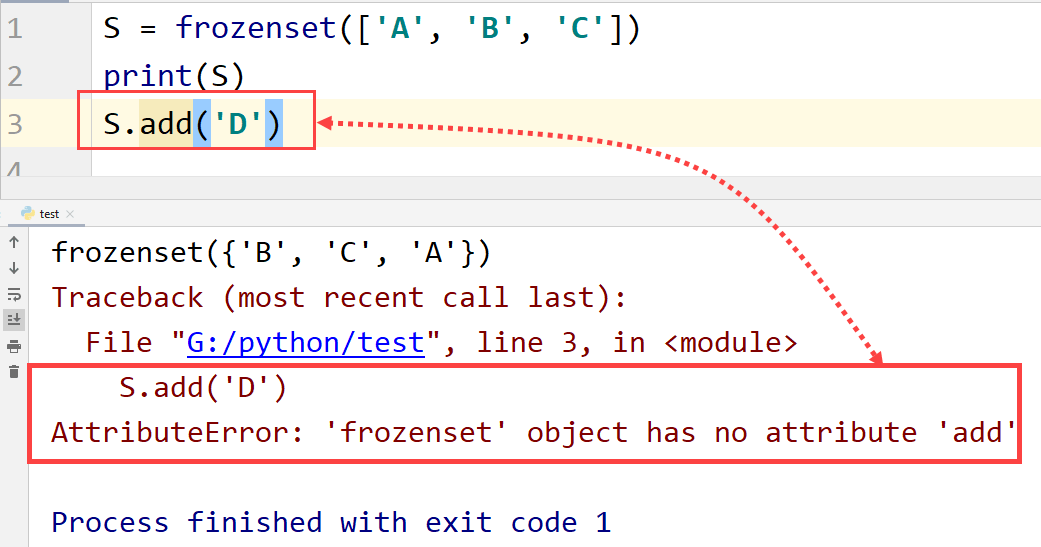## Frozen Sets

Python sets method
Method Description
clear() AND (Two Operands)This empties the whole Set, i.e., it removes all of the elements from the Set
min() It will return the smallest value in the Set.
max() It will return the largest value in the Set.
copy() This method will return a copy of the Set.
difference() It will return the difference between two Sets.
discard() This removes a specified element from the Set.
difference_update() Removes common elements between two sets from the Set on which the function is used.
pop() Removes an element from the set and returns it.
intersection() Returns the common elements from two Sets.
intersection_update() This will update the Set with intersection of some another Set.
isdisjoint() It returns True if two Sets have an empty intersection.
issubset() It returns True if a Set is a part of another Set.
issuperset() It returns True if a Set contains some another Set.
union() Returns all the elements that are present in two Sets.
update() It adds multiple items to a Set.
remove() It is used to remove items from a Set. It is similar to the discard() method.
all() This will return True if the Set is empty.
sorted() This will return a new list of Set elements that will be sorted.
symmetric_difference() It returns all the elements that are not common in two Sets.
sum() It will add all the elements in a Set and will return the sum.
enumerate() This will return an enumerate object. The output will contain the element and its index as a pair.
any() This will return False if the set is empty.
symmetric_difference_update() This will update a Set with the symmetric difference of two sets.